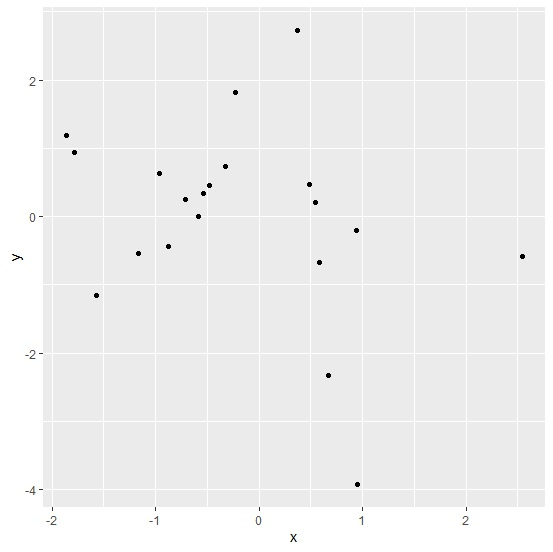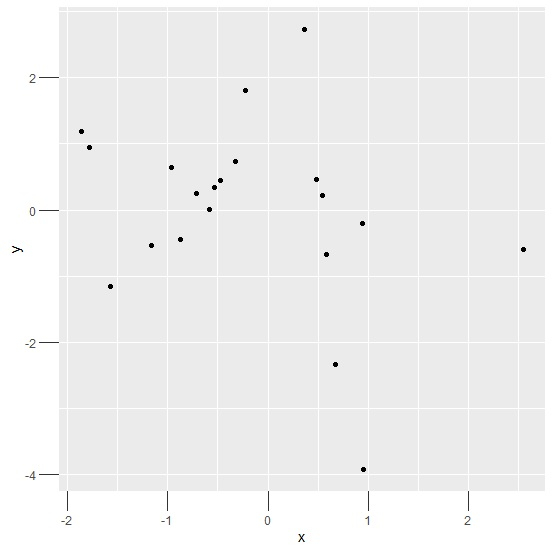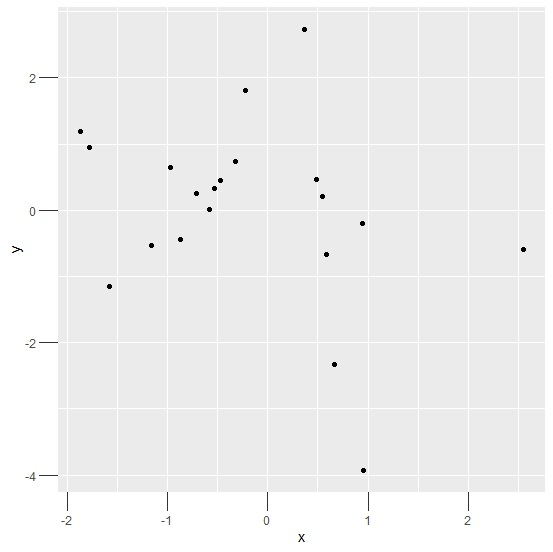# How to change the tick size using ggplot2 in R?

To change the tick size using ggplot2, we can use theme function with argument axis.ticks.length. For example, if we have a data frame called df that contains two columns say x and y then the scatterplot between x and y with larger size of tick marks can be created by using the below command −

ggplot(df,aes(x,y))+geom_point()+theme(axis.ticks.length=unit(0.8,"inch"))

## Example

Consider the below data frame −

Live Demo

x<-rnorm(20)
y<-rnorm(20)
df<-data.frame(x,y)
df

## Output

      x              y
1   2.5494830  -0.587904992
2  -0.5864972   0.006669457
3   0.9554755  -3.925291145
4   0.6686948  -2.326550523
5  -0.3267310   0.738981444
6  -0.8751193  -0.438232843
7   0.5477108   0.214512133
8  -0.4767738   0.451001999
9  -0.5364709   0.335897135
10   0.5853087 -0.665692002
11 -1.7818255   0.944527193
12 -0.9670748   0.639372414
13  0.4873353   0.466036727
14  0.3669196   2.732934553
15  0.9450203  -0.201909686
16 -1.1646289  -0.533447384
17 -1.8637459   1.186856615
18 -1.5751590  -1.154048208
19 -0.2266776   1.813758654
20 -0.7125976   0.251823020

Loading ggplot2 package and creating scatterplot between x and y with default size of tick marks −

## Example

library(ggplot2)
ggplot(df,aes(x,y))+geom_point()

## OutputCreating scatterplot between x and y with larger size of tick marks −

## Example

ggplot(df,aes(x,y))+geom_point()+theme(axis.ticks.length=unit(0.2,"inch"))

## Output## Example

ggplot(df,aes(x,y))+geom_point()+theme(axis.ticks.length=unit(0.50,"cm"))

## Output